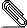Re: [Kwant] distinguishing bands belonging to different orbitals

Hi again Tibor,

>
> If I use your example code, in the step where
>
>
> the matrix H is diagonal in k-space, and would be block-diagonal in
> (electron,hole)-space, where each block is N-by-N (generally not
> diagonal), where N is the number of sites in the unit cell of a lead.
>
> However, KWANT seems to return H which is not in basis sorted as
> (electron,hole)-space, hence H is not block-diagonal.

Indeed, Kwant orders the Hilbert space first by site, and then by any
internal degrees of freedom (electron-hole in your case), so the
block-diagonal structure will not be obvious in this ordering.

> I would need to rearrange the basis that H is written in.
>
> Is there an easy way to do this?

If you want to get out just the electron or just the hole part of the
Hamiltonian you could construct a projector over the appropriate
orbitals. Assuming everything is ordered (electron, hole) then:

P_electron = np.kron(np.eye(n_sites), np.array([[1, 0], [0, 0]]))
P_hole = np.kron(np.eye(n_sites), np.array([[0, 0], [0, 1]]))

You can also (since Kwant 1.3) declare any discrete symmetry that your
model has by passing a 'conservation_law' when constructing your
Builder. In your case you would do something like:

TS = kwant.TranslationalSymmetry((-1, 0))
DS = np.diag([-1, 1]) # discrete symmetry operator with integer
eigenvalues
syst = Builder(TS, conservation_law=DS)
...
fsyst = syst.finalized()

After finalization you  can get the discrete symmetry over the whole
Hilbert space (of a single unit cell
for systems with a translational symmetry) by using the
'discrete_symmetry()' method of finalized systems:

sym = fsyst.discrete_symmetry()
P_electron, P_hole = sym.projectors

You can apply these projectors to the hamiltonian (they are just sparse
matrices) to get the corresponding
blocks:

H_electron = P_electron @ H @ P_electron.conjugate().transpose()
H_hole = P_hole @ H @ P_hole.conjugate().transpose()

Specifying 'conservation_law' and letting Kwant build the projectors for
you is simpler when your symmetry
operator is larger, and is not diagonal in the basis in which you write
the Hamiltonian when constructing the Builder.

Hope that helps,

Joesignature.asc
Description: OpenPGP digital signature